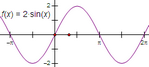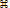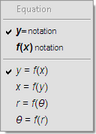# Plot Function/Plot New FunctionSelection prerequisites: One or more functions, or no objects

This Graph menu command plots one or more selected functions, or creates and plots a new function if no objects are selected.

The keyboard shortcut for Plot New Function is Ctrl+G (Windows) orG (Mac).

If you’re plotting a new function, the Calculator appears, just as it does for the Number | New Function command, to allow you to define the function to be plotted.

Once you’ve plotted a function, you can change the domain or the number of samples by using the Plot Properties dialog box. You can also change the domain by dragging the arrows at the ends of the function plot.You can change the equation of the function to plot it as a polar function r = f(θ), or to plot it with axes reversed, as x = f(y). To do so, double-click the function, or select it and choose When the Calculator appears, choose the equation you want from the Equation pop-up menu. You can also set the function's display to either y= notation or f(x) notation.